Follow

# Can I define a custom Risk Rating calculation?

Most project management methodologies including PMBOK and PRINCE2 suggest that you calculate a Risk Rating for every risk that is raised for a project. By default, PPO is configured to support the calculation of risk ratings using the models suggested by PMBOK and PRINCE2 which incorporates the Risk Impact and Probability.

Some organisations, however, have more complex requirements which incorporate other factors and also apply weightings to these factors.  The custom list item weighting feature is ideally suited for this.

The standard configuration

By default, PPO uses the sort order of the items in the Risk Impact and Risk Probability custom list items to calculate the Risk Rating. Below you can see the details of the default Risk Impact and Risk Probability custom lists.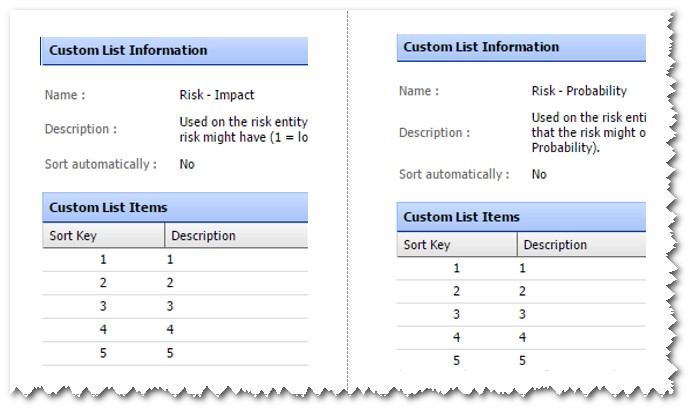Therefore, if a risk has a Probability of 4 and an Impact of 1, the Rating will be 4 as in the screenshot below.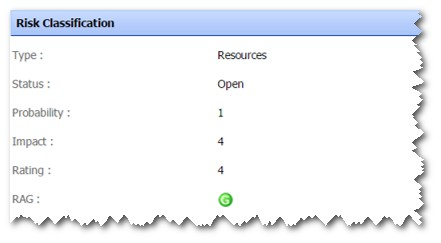Note that in this case the descriptions of the items are the same as the sort order, but this is not required.  For instance, instead of using a value of "5", you could change the description of the item to "Very High".  The rating calculation would still work since it uses the Sort Key.

The above default configuration means that the lowest Rating will be 1 (when the Probability and Impact are both 1) and the highest Rating is 25 (when the Probability and Impact are both 5).

Defining a custom, weighted risk rating calculation

Although the default risk model as described above aligns to PMBOK, some customers have their own risk models. Also, sometimes the model requires that you implement different weightings when calculating the risk rating. We will use the model below as an example: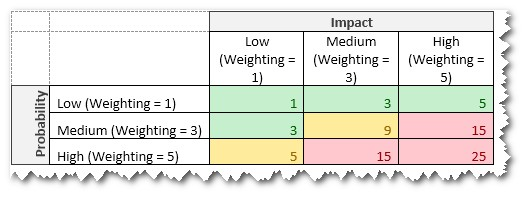With the custom list item weighting functionality this can easily be accommodated without requiring that the PPO support team create a custom risk rating function for you.

The first step is to change the Risk Impact and Probability custom lists as shown below: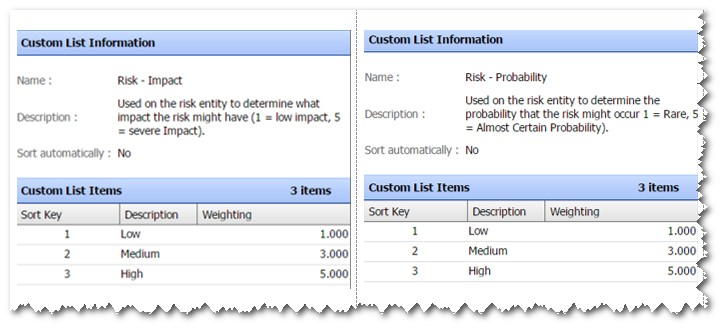As can be seen from the screenshot above, a weighting has been specified for each custom list item as per the model.

In order to calculate the Rating based on the model previously described, we change the formula for the RiskRating field as shown in the screenshot below: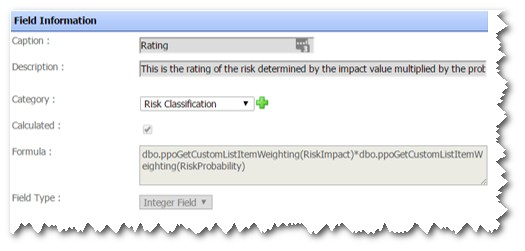Note that since this is a standard field you will have to log a support ticket in order to have the formula changed as above. If you however subsequently want to change the weightings, you can do this yourself by updating the weightings on the custom list items.

Have questions?